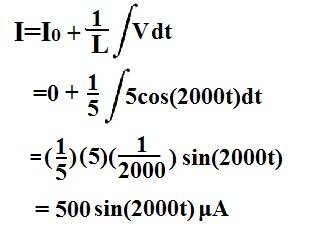﻿ How to Calculate the Current Through an Inductor

# How to Calculate the Current Through an InductorTo calculate the current going through an inductor, the formula below is used:All you must know to calculate the current going through an inductor is L, the inductance of the inductor and the integral of the voltage that is across the inductor.

Note: Io is the initial current going through the inductor, if there is any.

Example
If the voltage across an inductor is 5cos(2000t)V and the inductance of the inductor is 5μH, what is the current going through the inductor?
(Initial Conditions:Io=0A)